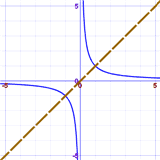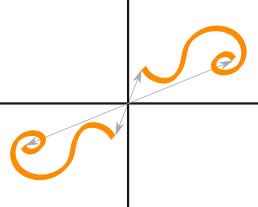# Symmetry in Equations

Equations can have symmetry:Graph of x2Graph of 1/x

Diagonal symmetry

In other words, there is a mirror-image.

## Benefits

The benefits of finding symmetry in an equation are:

• we understand the equation better
• it is easier to plot
• it can be easier to solve. When we find a solution on one side, we can then say "also, by symmetry, the (mirrored value)"

## How to Check For Symmetry

We can often see symmetry visually, but to be really sure we should check a simple fact:

Is the equation unchanged when using symmetric values?

How we do this depends on the type of symmetry:

For symmetry with respect to the Y-Axis, check to see if the equation is the same when we replace x with x:

### Example: is y = x2 symmetric about the y-axis?

Try to replace x with x:

y = (−x)2

Since (−x)2 = x2 (multiplying a negative times a negative gives a positive), there is no change

So y = x2 is symmetric about the y-axis

Use the same idea as for the Y-Axis, but try replacing y with y.

### Example: is y = x3 symmetric about the x-axis?

Try to replace y with y:

−y = x3

Now try to get the original equation:

Try multiplying both sides by 1:

y = −x3

It is different.

So y = x3 is not symmetric about the y-axis

### Diagonal Symmetry

Try swapping y and x (i.e. replace both y with x and x with y).

### Example: does y = 1/x have Diagonal Symmetry?

y = 1/x

Try swapping y with x:

x = 1/y

Now rearrange that: multiply both sides by y:

xy = 1

Then divide both sides by x:

y = 1/x

And we have the original equation. They are the same.

So y = 1/x has Diagonal Symmetry

### Origin SymmetryOrigin Symmetry is when every part has a matching part:

• the same distance from the central point
• but in the opposite direction.

Check to see if the equation is the same when we replace both x with x and y with y.

### Example: does y = 1/x have Origin Symmetry?

y = 1/x

Replace x with x and y with y:

(−y) = 1/(−x)

Multiply both sides by 1:

y = 1/x

And we have the original equation.

So y = 1/x has Origin Symmetry

Amazing! y = 1/x has origin symmetry as well as diagonal symmetry!

1154, 1155, 2463, 2464, 2465, 4042, 4043, 4044, 4045, 4046